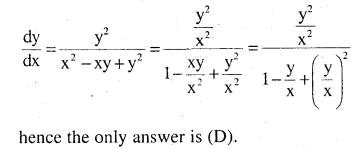# 2nd PUC Maths Question Bank Chapter 9 Differential Equations Ex 9.5

Students can Download Maths Chapter 9 Differential Equations Ex 9.5 Questions and Answers, Notes Pdf, 2nd PUC Maths Question Bank with Answers helps you to revise the complete Karnataka State Board Syllabus and score more marks in your examinations.

## Karnataka 2nd PUC Maths Question Bank Chapter 9 Differential Equations Ex 9.5

### 2nd PUC Maths Differential Equations NCERT Text Book Questions and Answers Ex 9.5

In each of the Exercises 1 to 10, show that the given differential equation is homogeneous and solve each of them.

Question 1.
(x2 + xy) dy = (x2 + y2) dx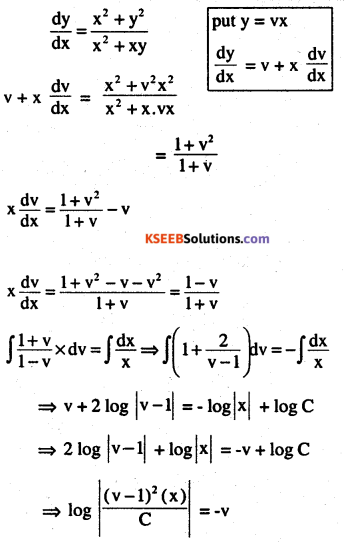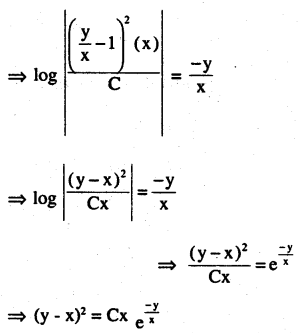Question 2.
$$y^{\prime}=\frac{x+y}{x}$$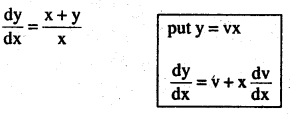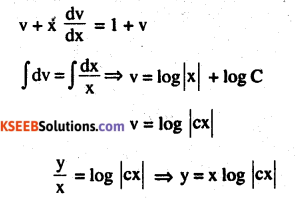Question 3.
(x- y)dy – dy -(x+y)dx = o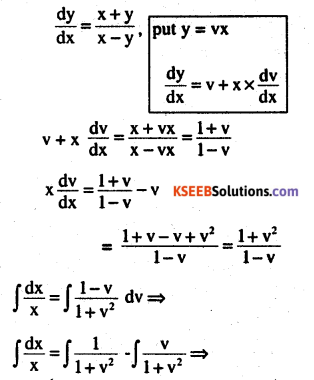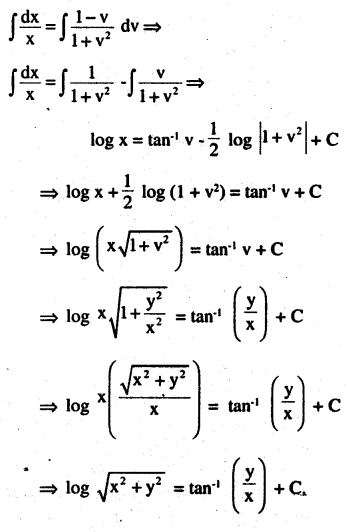Question 4.
(x2 – y2) dx + 2xy dy =0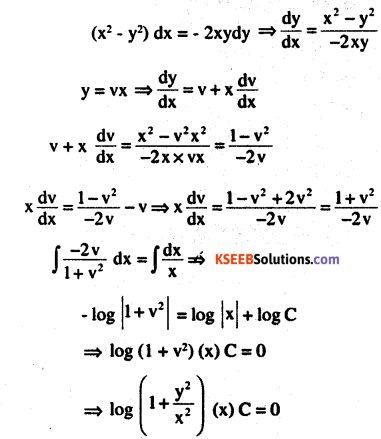Question 5.
$$x^{2} \frac{d y}{d x}=x^{2}-2 y^{2}+x y$$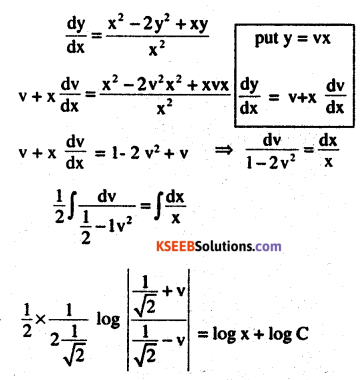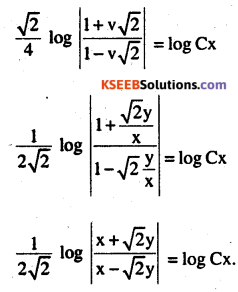Question 6.
$$\mathbf{x} \mathrm{d} \mathbf{y}-\mathbf{y} \mathbf{d x}=\sqrt{\mathbf{x}^{2}+\mathbf{y}^{2}} \mathbf{d} \mathbf{x}$$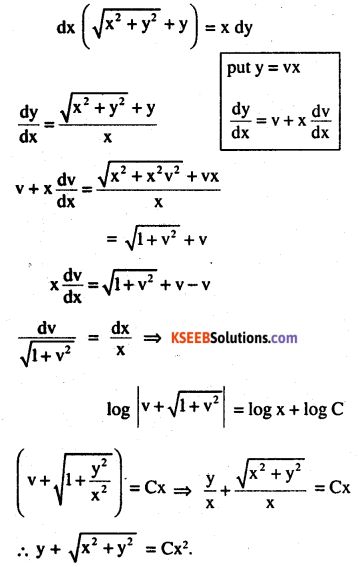Question 7.
$$\left\{x \cos \left(\frac{y}{x}\right)+y \sin \left(\frac{y}{x}\right)\right\} y d x$$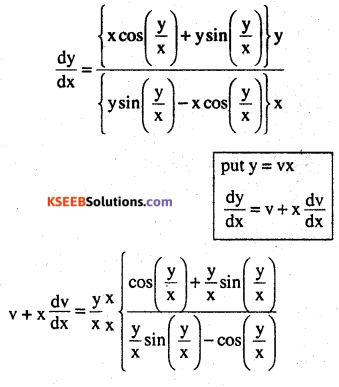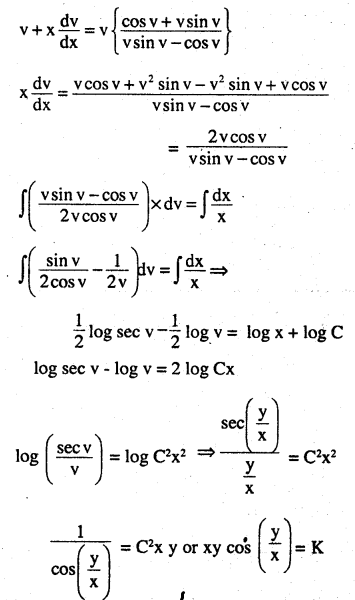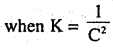Question 8.
$$x \frac{d y}{d x}-y+x \sin \left(\frac{y}{x}\right)=0$$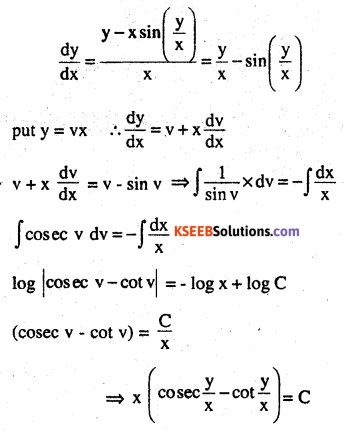Question 9.
$$y d x+x \log \left(\frac{y}{x}\right) d y-2 x d y=0$$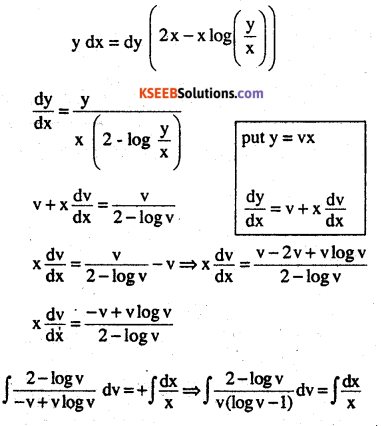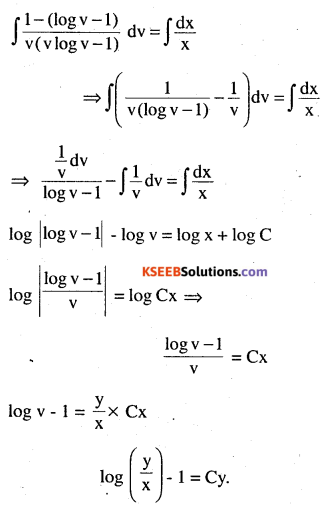Question 10.
$$\left(1+e^{\frac{x}{y}}\right) d x+e^{\frac{x}{y}}\left(1-\frac{x}{y}\right) d y=0$$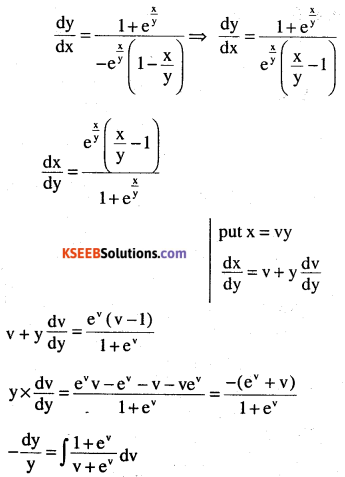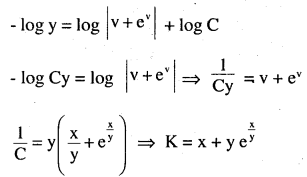For each of the differential equations in Exercises from 11 to 15, find the particular solution satisfying the given condition:

Question 11.
(x + y) dy + (x – y) dx = 0; y = 1 when x = 1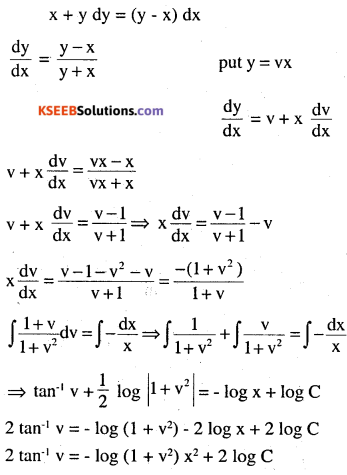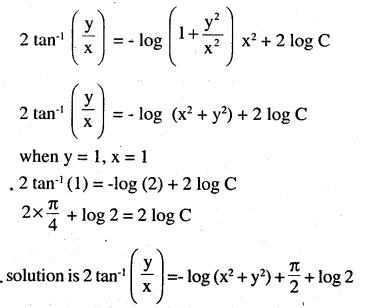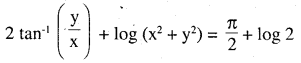Question 12.
x2dy + (xy + y2) dx = 0; y = 1 when x = 1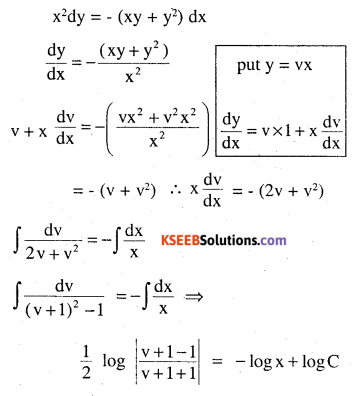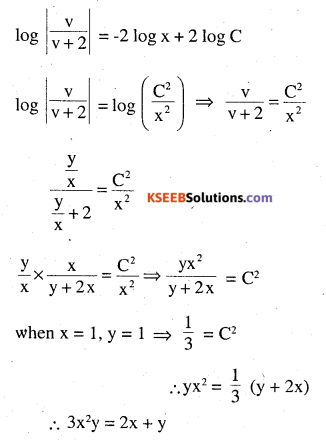Question 13.
$$\left[x \sin ^{2}\left(\frac{y}{x}\right)-y\right] d x+x d y=0 ; y=\frac{\pi}{4}$$ when x = 1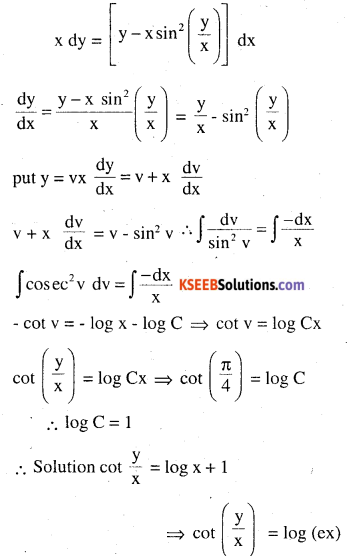Question 14.
$$\frac{d y}{d x}-\frac{y}{x}+\csc \left(\frac{y}{x}\right)=0 ; y=0 \text { when } x=1$$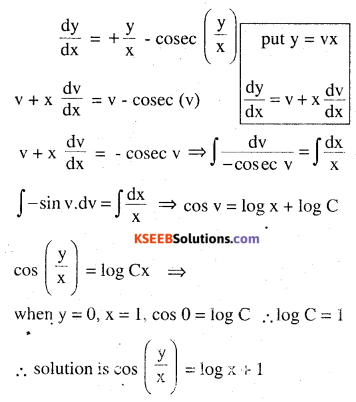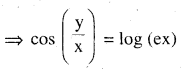Question 15.
$$2 x y+y^{2}-2 x^{2} \frac{d y}{d x}=0 ; y=2 \text { when } x=1$$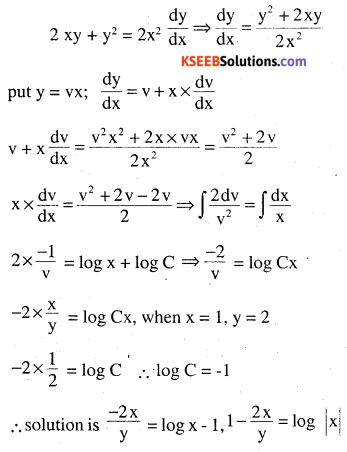Question 16.
A homogeneous differential equation of the from can be solved by making the subtitution.
(A) y = vx
(B) v = yx
(C) x = vy
(D) x = v
$$\frac{x}{y} \text { or } \frac{y}{x}$$